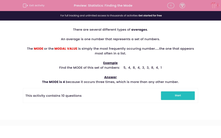# Statistics: Finding the Mode

In this worksheet, students calculate the mode of a given set of data.Key stage:  KS 2

Curriculum topic:   Maths and Numerical Reasoning

Curriculum subtopic:   Mean, Median, Mode and Range

Difficulty level:#### Worksheet Overview

There are several different types of averages.

An average is one number that represents a set of numbers.

The MODE or the MODAL VALUE is simply the most frequently occuring number.....the one that appears most often in a list.

Example

Find the MODE of this set of numbers:    5,  4,  8,  4,  3,  3,  8,  4,  1